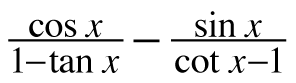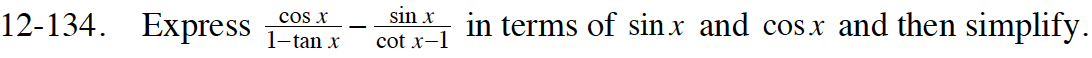Home > CCA2 > Chapter 12 > Lesson 12.2.3 > Problem12-134

12-134.

Expressin terms of sin x and cos x and then simplify. Homework Help ✎How can you change tan(x) and cot(x) to sin(x) and cos(x)?
What ratios using those trig functions do they equal?

Simplify create common denominators in the denominators of the larger fractions.

Simplify each fraction using a Giant One.

Notice you have created a common denominator.
Combine the two fractions.
The numerator is a difference of two squares.
Keep simplifying.

$\frac{\cos(x)}{1-\frac{\text{sin}(x)}{\text{cos}(x)}}-\frac{\sin(x)}{\frac{\text{cos}(x)}{\sin(x)}-1}$

$\frac{\cos \left(x\right)}{\frac{\cos \left(x\right)-\sin \left(x\right)}{\cos \left(x\right)}}-\frac{\sin \left(x\right)}{\frac{\cos \left(x\right)-\sin \left(x\right)}{\sin \left(x\right)}}$

$\frac{\cos \left(x\right)}{\cos \left(x\right)}\cdot \frac{\cos \left(x\right)}{\frac{\cos \left(x\right)-\sin \left(x\right)}{\cos \left(x\right)}}-\frac{\sin \left(x\right)}{\frac{\cos \left(x\right)-\sin \left(x\right)}{\sin \left(x\right)}}\cdot \frac{\sin \left(x\right)}{\sin \left(x\right)}$

$\frac{\cos ^2\left(x\right)}{\cos \left(x\right)-\sin \left(x\right)}-\frac{\sin ^2\left(x\right)}{\cos \left(x\right)-\sin \left(x\right)}$